# Physics - Laws of Traverse Vibrations

Laws of Traverse Vibrations

The fundamental frequency of a stretched String fixed at both end is given by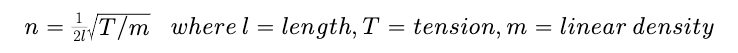First Law or Law of Length :

When the tension (T) and linear density (m) are constant, the frequency of Vibration (n) of a streched String vibrating in one segment is inversely proportional to its length (l)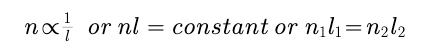Second Law or Law of Tensions :

When the length (l) and the linear density (m) are constant, the frequency of Vibration (n) of a streched String vibrating in one segment is inversely to the square root of the tension (T) in the wire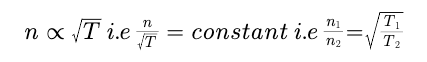Third Law or Law of Linear Densities :

When the length (l) and Tension (T) are constant, the frequency of a streched string vibrating in one Segment is inversely proportional to square root of linear density.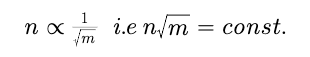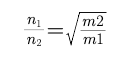Post By : Ashish Maurya 17 May, 2020 317 views Physics